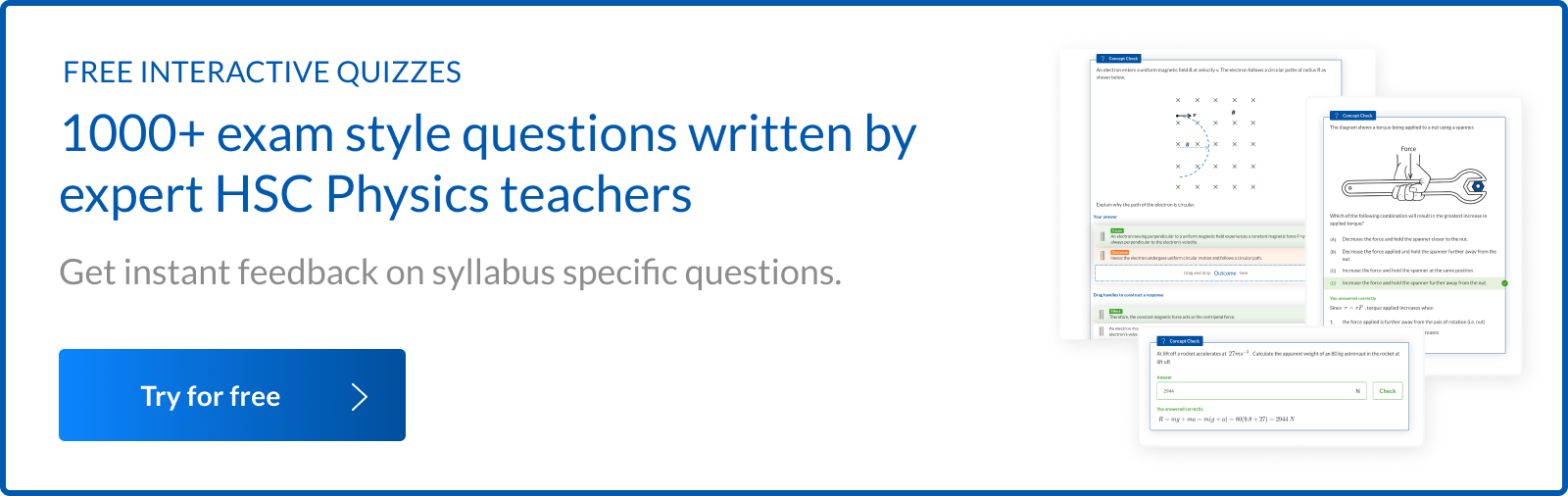# 20 Must Know HSC Physics Questions before your HSC Physics Exam

Need HSC exam questions based on the new HSC Physics Syllabus? Here are 20 HSC Physics questions Learnable Founder DJ Kim predicts may be in the 2019 HSC Exam.

## Are you wondering what will be in this year’s HSC Physics Exam?

Like other Year 12 Physics students, you’ve probably been frustrated by the lack of past HSC Physics exam papers available to guide your preparation for the upcoming HSC Physics Exam.

However, there is an advantage of the being the first cohort for sitting the HSC Physics Exam based on the new syllabus:

The exam questions are going to be a lot more predictable than you think.

Let’s examine the new HSC Physics topics introduced and the new HSC Physics exam questions that are likely to appear in this year’s HSC Physics Exam.

## New Topics introduced in 2019 HSC Physics Syllabus

2019 HSC Physics syllabus introduced new topics over the four modules (Modules 5 – 8). Understanding the new changes will ensure that you are not misguided by the previous HSC exam questions based on the old syllabus.

New topics introduced in ‘Module 5 Advanced Mechanics’ in the new 2019 syllabus are outlined below:

 New topics introduced the 2019 HSC Physics Syllabus New Topics Key Concepts HSC Physics Question Types Circular Motion Uniform circular motion in the horizontal and vertical planes Quantitative analysis of:Car on banked track with no frictionThe conical pendulumA spinning wheel or discFerris wheel Non uniform circular motion Quantitative and qualitative analysis of Loop-de-loop maneuver Kepler’s Laws Kepler’s first and second laws Qualitative analysis of motion of objects in terms of Kepler’s first and second laws. Energy of orbits Energy of a satellite in orbit Quantitative analysis of:Total energy of a satellite in orbitWork required to go into orbitEnergy required to change orbits

### Module 6: Electromagnetism

New topics introduced in ‘Module 6 Electromagnetism’ from the new 2019 HSC Physics syllabus are outlined below:

 New topics introduced the 2019 HSC Physics Syllabus Topic Key Concept HSC Physics Question Types Magnetic field Magnetic field produced by a conductor Quantitative analysis of magnetic field produced by a long straight current carrying conductorQuantitative analysis of magnetic field produced by a solenoid Electromagnetic Induction Faraday’s Law of electromagnetic induction Quantitative analysis of induced emf from a change in magnetic flux

### Module 7: The Nature of Light

The new topics introduced in ‘Module 7 The Nature of Light’ in the new 2019 HSC Physics syllabus are outlined below:

 New topics introduced the 2019 HSC Physics Syllabus New Topics Key Concepts HSC Physics Question Types Wave Model of Light Thomas Young’s double slit experiment Hugyens’ wave model vs Newton’s particle model of lightQualitative & quantitative analysis of Thomas Young’s double slit experiment Polarisation of transverse waves Using Malus’s Law to calculate the intensity of polarised light Measurements of the Speed of Light Types of measurements of speed of light Astronomical measurementsTime of flight measurements Spectroscopy Atomic & Stellar Spectra Spectra of elements and compoundsQualitative analysis of different light sourcesSpectra of starsDoppler shift & translations velocity of starDoppler broadening & rotational velocity of starPressure broadening & density of gas Special Relativity Relativistic momentum and mass dilation Quantitative analysis of relativistic momentum and mass dilationExplaining the consequences of mass dilation

### Module 8: From the Universe to the Atom

The new topics introduced in ‘Module 8 From the Universe to the Atom’ in the new 2019 HSC Physics syllabus are outlined below:

 New topics introduced in the 2019 HSC Physics Syllabus New Topics Key Concepts HSC Physics Question Types Millikan’s oil drop experiment Charge of an electron Qualitative and quantitative analysis of the results of the oil drop experiment Schrodinger’s contribution Schrodinger’s equation and the model of the atom Discussing Schrodinger’s contribution to atomic theory Nuclear stability and decay Half life and activity Quantitative analysis of a sample of radioactive substanceHalf life and radioactive decay constant Expansion of the universe Cepheid variable stars and Hubble’s law Discussing the significance of Cepheid variable stars in cosmologyDescribing the process for discovering the expansion of the universe by Edwin Hubble The Big Bang Theory Evolution of the universe Describing the evidence for the Big BangDescribing the process of accretion of stars and galaxies Classification of stars Hertzsprung-Russell diagrams Describing the relationship between the mass of stars and their luminosities and lifetime.Comparing the energy production processes of Main Sequence stars and Red Giants or White Dwarfs Stellar evolution Evolutionary stages for a star Explaining the initial stage of formation of a starExplaining the evolutionary stages for a star with 1 -3 solar mass

## New HSC Physics Exam Questions on ‘Advanced Mechanics’

### Question 1: Conical Pendulum

In a conical pendulum, a bob of mass 100 g is attached to a fixed point by a string of length 0.5 m and is rotating with constant speed in a horizontal circle of radius 0.3 m.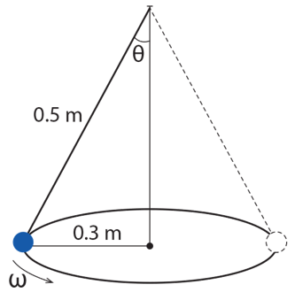(a) Show that the acceleration of the bob is given by $a = gtan\theta$ 2 marks (b) Calculate the angular speed of the bob. Express your answer to two significant figures. 3 marks (c) Hence or otherwise, calculate the centripetal force acting on the bob. Express your answer to two significant figures 2 marks

See Question 1 solution.

### Question 2: Banked Track

A moving cyclist is perpendicular to a banked circular track which is 40° from horizontal, as pictured below: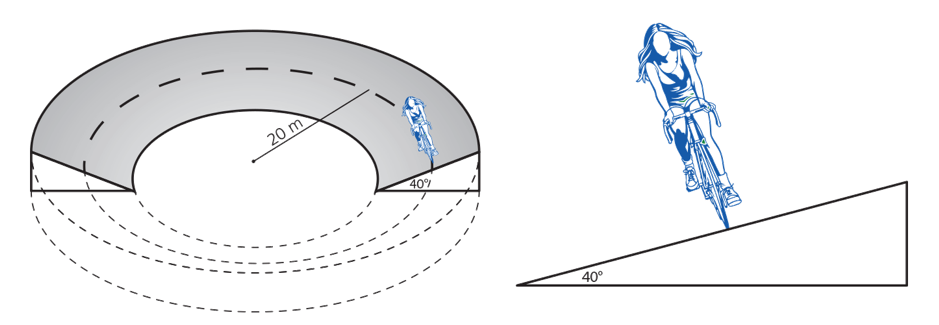The cyclist is travelling around the circular track at a radius of 20 m.

 (a) Find the components of force on the cyclist and relate these to centripetal force on the cyclist. 2 marks (b) Use the equations in part (a) to derive an expression for the speed of the cyclist and calculate the speed of the cyclist. 3 marks

See Question 2 solution.

### Question 3: Ferris wheel

The London Eye is the fourth largest Ferris wheel in the world. The wheel has a radius of 135 m and rotates once every 30 minutes. Each of the 32 capsules has a mass of 32 tonnes. A schematic representation of the London Eye is shown below.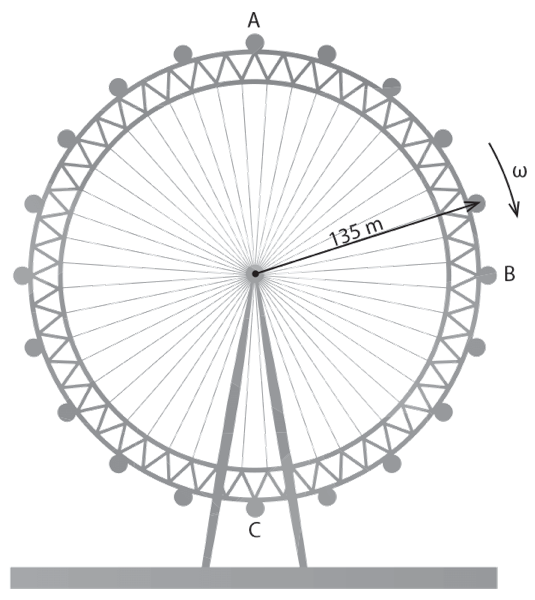(a) Compare qualitatively the speed of carriage A, B and C. 1 mark (b) Calculate the centripetal acceleration. 2 marks (c) Calculate the force that needs to be applied to the carriage at C to keep it rotating. 2 marks (d) Comment on how the magnitude of the force in (c) change compared to when the Ferris wheel is stationary. 1 mark

See Question 3 solution.

### Question 4: Non-uniform circular motion

NASA takes its astronauts to zero gravity flights using Boeing 727 jets. The aircraft gives its passengers the sensation of weightlessness by following a parabolic flight path at an altitude of 10 000 m above the Earth’s surface.

At the top of the flight, the trajectory can be modelled as an arc of a circle. A typical trajectory is shown in the diagram below.

###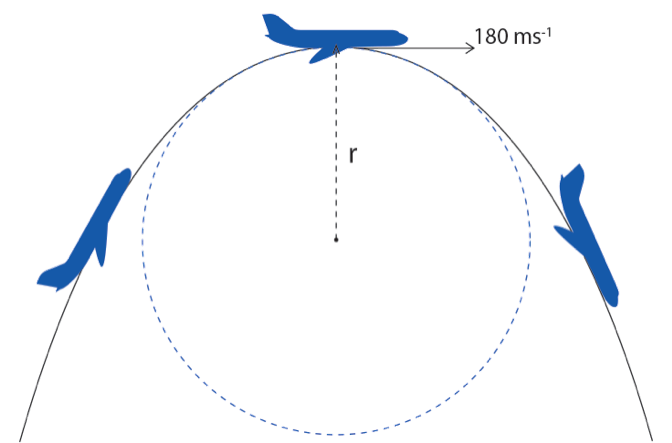(a) Calculate the radius of the arc that would give passengers zero gravity at the top of the flight if the jet is travelling at $180 \ ms^{-1}$ . Show your working 2 marks (b) Is the force of gravity on a passenger zero at the top of the flight? Explain what ‘zero gravity experience’ means 3 marks

See Question 4 solution.

### Question 5: Kepler’s Second Law

The orbit of Halley’s comet is shown below.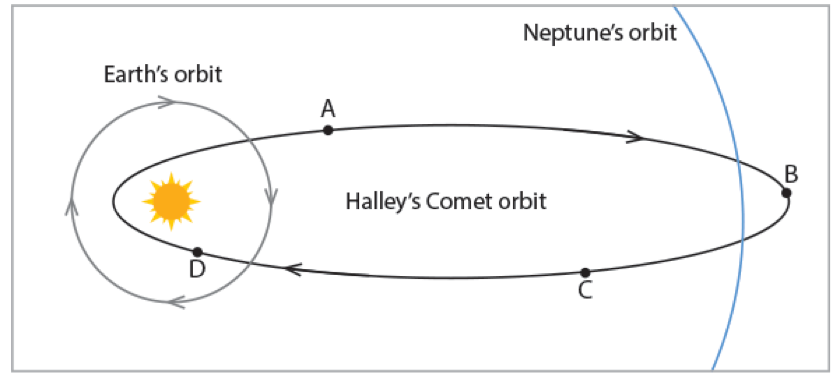(a) At which position is Halley’s comet moving the slowest? Give a reason for your answer. 2 marks (b) Explain how the motion of the Halley’s comet in its orbit supports Kepler’s second law. 3 marks

See Question 5 solution.

## New HSC Physics Exam Questions on ‘Electromagnetism’

### Question 6: Magnetic field strength of a solenoid

Two identical solenoids are positioned in a line as shown below. A current of 2 A flows through the coils and produces magnetic fields.

Ignore the effects of Earth’s magnetic fields.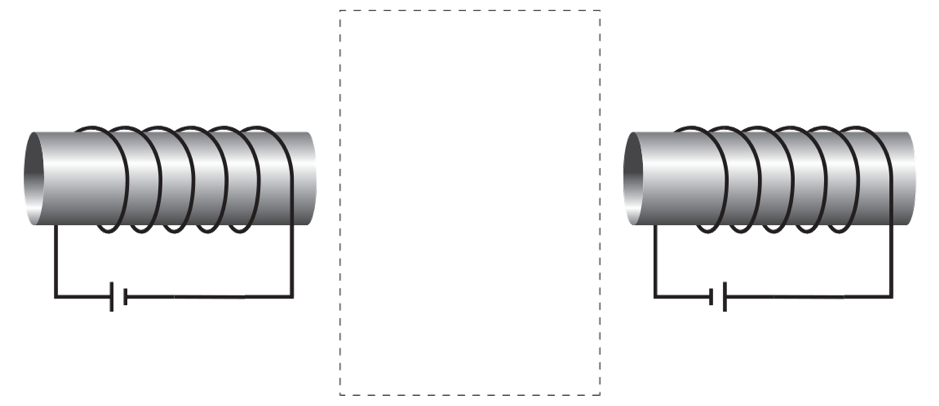(a) Sketch at least four field lines in the space enclosed by the dotted line. Clearly indicate the direction of each field line. 2 marks (b) Calculate the magnetic field strength produced by each solenoid.N = 6 turnsI = 2 AL = 20 cm 2 marks

See Question 6 solution.

### Question 7: Electromagnetic induction

A rigid metal rod AB is mounted on a rotating stand on a horizontal table. The rod rotates in a horizontal circle at constant speed in uniform magnetic field directed downward as shown in the diagram.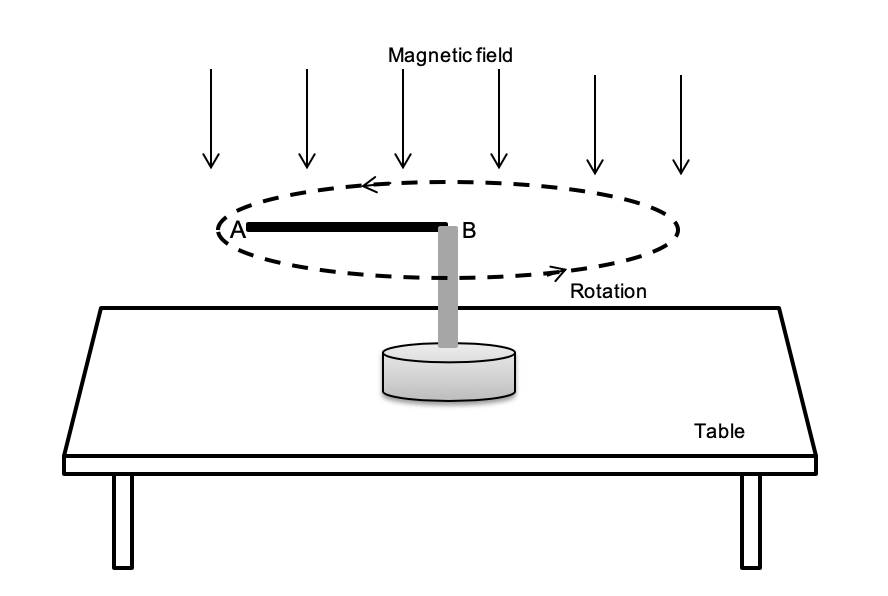(a) Which end of the rod is negative? 1 mark (b) Explain how the EMF is produced in the rod. 3 marks (c) Sketch a graph of induced emf versus time for two rotations.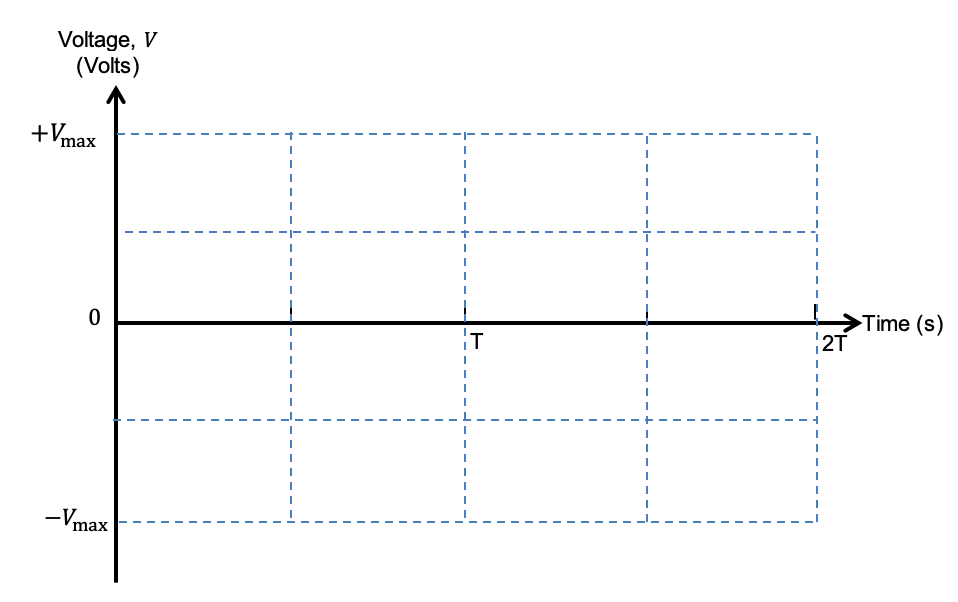2 marks

See Question 7 solution.

### Question 8: Quantitative analysis of Faraday’s Law of electromagnetism

A circular coil of 100 turns with a radius of 2.0 cm is placed in a changing magnetic field. The angle between the magnetic field lines and the plane of the coil is 90 degrees. The graph below shows the variation with time of the magnetic field strength.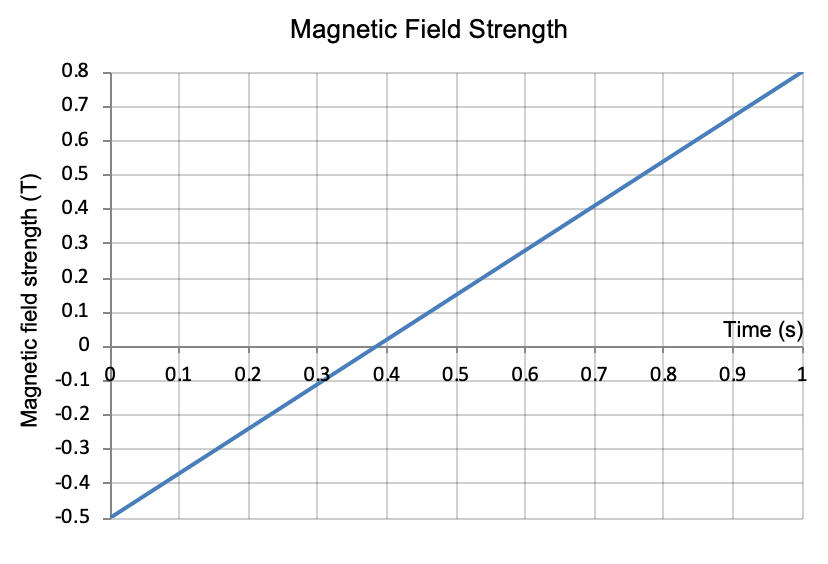(a) From the graph, calculate the change in magnetic flux experienced by the coil over the 1 second period. 2 marks (b) Calculate the magnitude of the induced emf in the coil. 2 marks

See Question 8 solution.

## New HSC Physics Exam Questions on ‘The Nature of Light’

### Question 9: Thomas Young’s Double Slit Experiment

An experimental setup to demonstrate Young’s double slit experiment is shown below.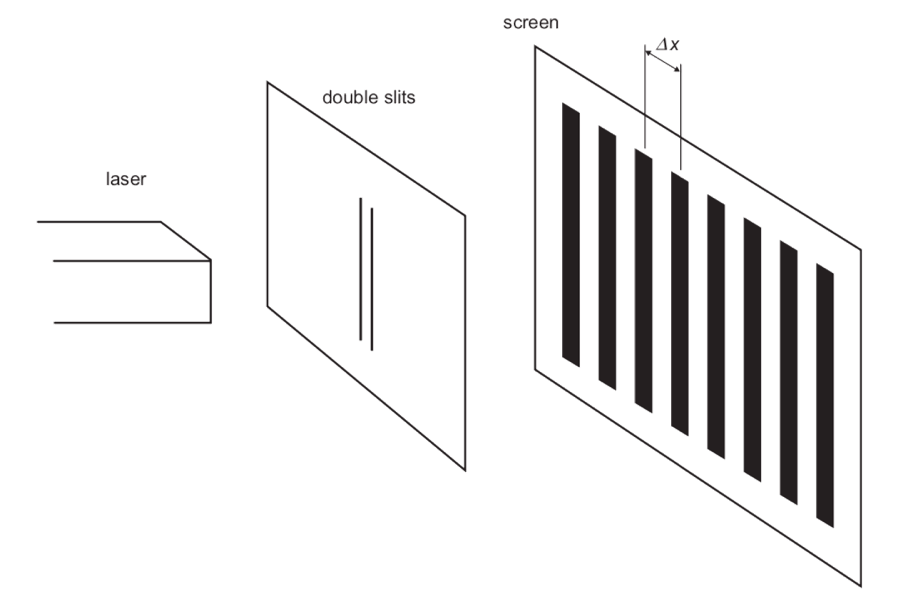A pattern of bright and dark bands is observed on the screen. Explain two changes that will increase the distance, Δx, between dark bands in this double slit interference pattern. (3 marks)

See Question 9 solution.

### Question 10: Polarisation of transverse waves

An unpolarised beam of light passes through a series of polarisers as shown below.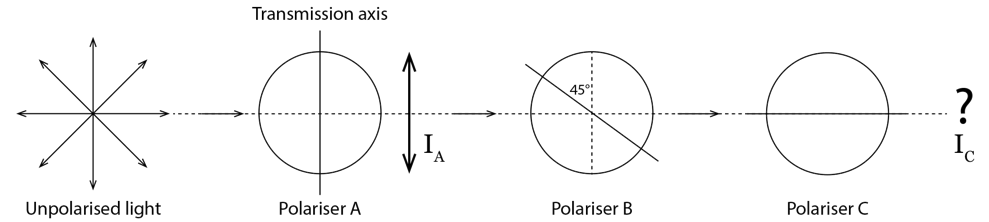Calculate the ratio of the intensities of the transmitted light, Ito IA. (2 marks).

See Question 10 solution.

### Question 11: Time of flight measurements

In the 1840s, French physicist, Hippolyte Fizeau performed an experiment to measure the speed of light.

Describe the method he used to determine the speed of light. You may use a diagram to assist your answer.  (4 marks)

See Question 11 solution.

### Question 12: Spectra of different light sources

The spectra of light from two different light sources are shown in the diagram below. The dashed lines indicate the range of visible wavelengths.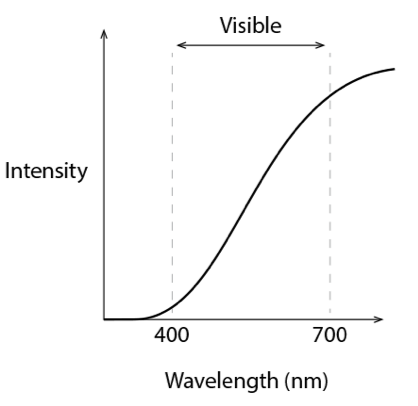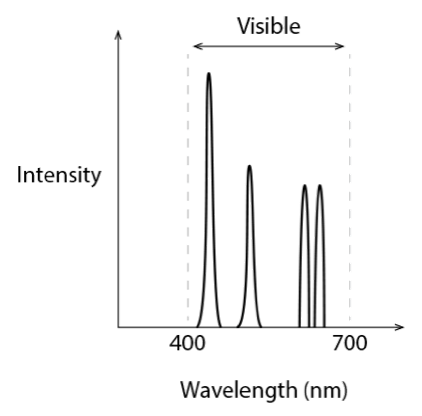Spectrum A Spectrum B

Four possible light sources are listed below:

• Blue laser light
• Mercury vapour lamp (discharge tube filled with Mercury vapour)
• 100 W incandescent globe
• Sunlight

Identify the light source for Spectrum A & B. Give reason for your answer. (4 marks)

See Question 12 solution.

### Question 13: Spectra of stars

The diagram below shows absorption spectra of a certain element from a discharge tube and two stars, A and B. Stars A and B are known to be stationary in space relative to the Earth.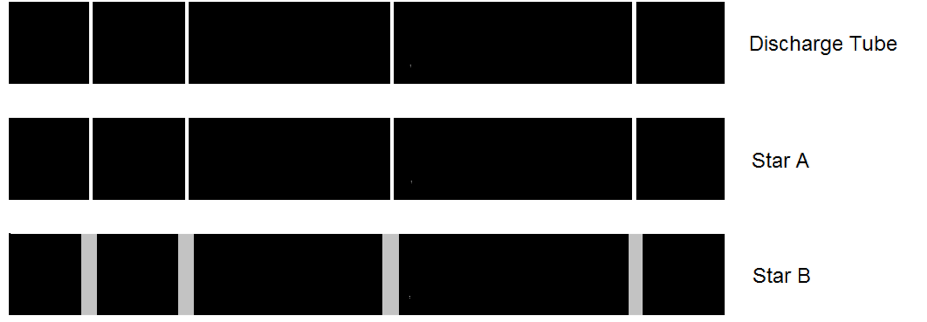(a) Outline the rotational motion of each star, giving reasons for your answer. 2 marks (b) Outline how the Doppler effect gives information about the translational motion of a star. 2 marks

See Question 13 solution.

### Question 14: Hafele-Keating experiment

The Hafele-Keating experiment is a famous experiment where three atomic clocks were synchronised and two were then flown around the world – one with the Earth’s rotation, and one in the opposite direction – while the third remained on the ground.

 (a) State the results of the experiment. 2 marks (b) Outline how the results from this experiment support the theory of Special Relativity. 3 marks

See Question 14 solution.

## New HSC Physics Exam Questions on ‘From the Universe to the Atom’

### Question 15: Millikan’s oil drop experiment

Millikan and Fletcher’s determined the charge of an oil drop.

 (a) Outline how the charge was measured. 3 marks (b) State the conclusion of their experiment 1 mark

See Question 15 solution.

### Question 16: Schrodinger’s contributions to atomic theory

Describe how contributions to atomic theory made by Schrodinger changed the de Broglie-Bohr model of the atom. (4 marks)

See Question 16 solution.

### Question 17: Half-life & decay constant

Technetium-99m is a commonly used medical radioisotope. It is a gamma emitter and is used for diagnosing a number of bone conditions.

The graph below shows the decay rate for a radioisotope in the blood of a patient after a nuclear medicine procedure.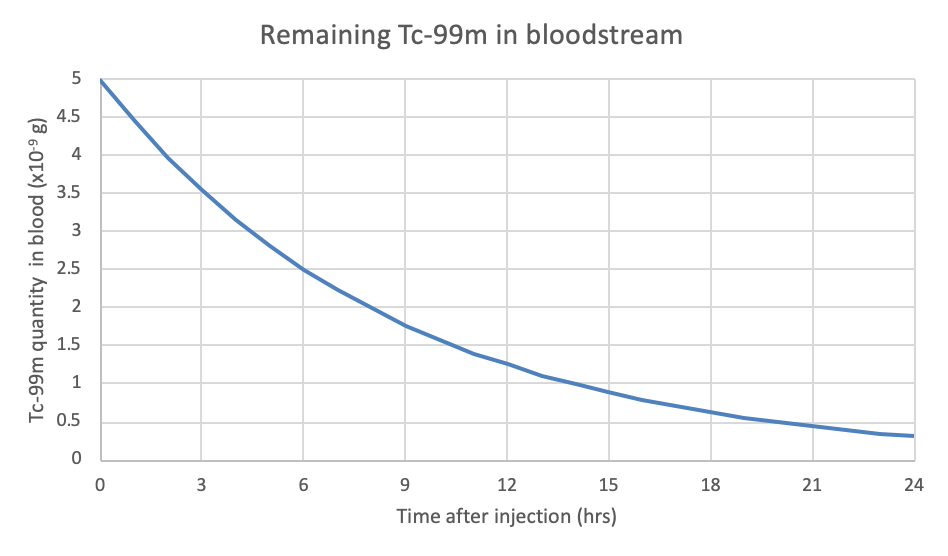(a) What is the half-life of Technetium-99m? 1 mark (b) Calculate the decay constant λ for technetium-99m. 2 marks

See Question 17 solution.

### Question 18: Hubble’s Law

Hubble provided observation proof that the universe was expanding and supported the prediction of Friedmann of an expanding universe.

Explain how he used cosmic redshift observations and Cepheid variable stars to provide the observational proof. (5 marks)

See Question 18 solution.

### Question 19: Hertzsprung-Russell Diagram

A possible evolutionary path of a star is shown on the Hertzsprung-Russell (H-R)  diagram.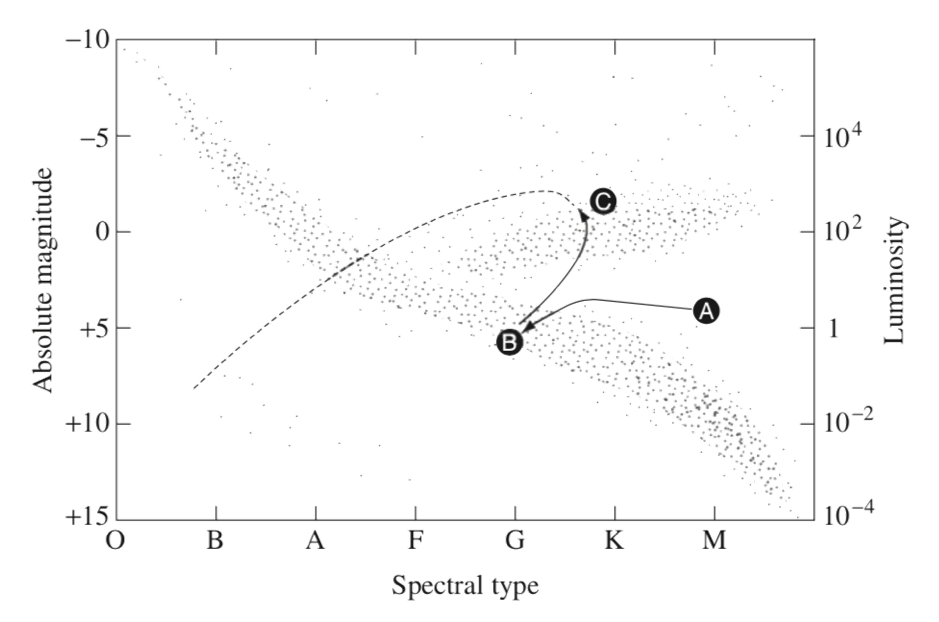(a) Which of the stars is producing the greatest amount of light? Give a reason for your answer. 2 marks (b) Which star has the lowest surface temperature? Give a reason for your answer. 2 marks

See Question 19 solution.

### Question 20: Evolutionary stages for a star

The evolutionary tracks of two stars, X and Y, are shown in the diagram below.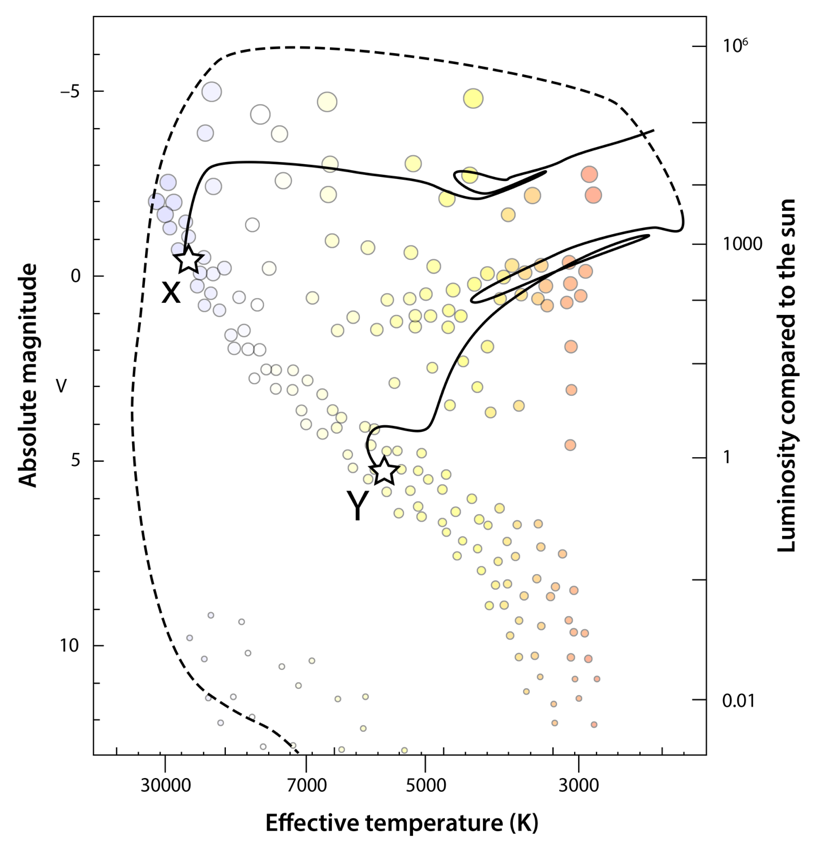(a) Briefly explain the initial stage of formation of a star. 2 marks (b) Which star, X or Y, has the larger mass? Give a reason for your choice. 2 marks (c) Explain why a star ten times more massive than the Sun stays on the main sequence for a much shorter time than a star of 1 solar mass 2 marks (d) The evolution of star Y after it leaves the main sequence can be summarised as follows:Red giant → Planetary nebula → White dwarfDescribe the properties of Red giant and White dwarf in terms ofsurface temperature relative to the sunluminosity relative to the sunnuclear reactions relative to the sun 3 marks

See Question 20 solution.

## Solution

 Q Answer 1 (a) Analyse the forces acting parallel and perpendicular to acceleration. $$T\sin\theta = ma \ and \ T\cos\theta =mg$$ $$\therefore \frac{T\sin\theta}{T\cos\theta} = \frac{ma}{mg} \\ tan\theta = \frac{a}{g} \\ a = \tan\theta$$(b) Find an expression for ω and then calculate.Step 1: Find the expression for tan θ the string makes with vertical $$tan\theta = \frac{r}{h}=\frac{3}{4}$$Step 2: Find an expression for ω $$a = g\tan\theta \\ r\omega^2 = g\tan\theta \\ \omega =\sqrt{\frac{gtan\theta}{r}} \\ \omega = \sqrt{\frac{9.8 \times \frac{3}{4}}{0.3}} \\ \omega = 5.0 rad/s$$ (c) The centripetal force is given by $$F_c=mr\omega^2$$$$F_c=0.1 \times 0.3 \times 5.0^2 = 0.75 N$$ 2 (a) The force diagram is shown below.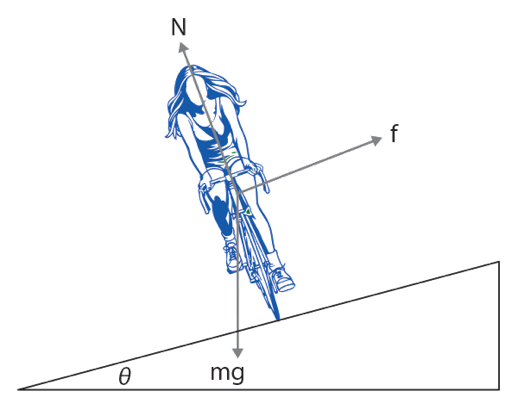The components of the normal force are:Horizontal component: NcosθVertical component:  Nsinθ.The horizontal component is in the same direction as the centripetal acceleration: $$N\sinθ = \frac{mv^2}{r}$$(b) Analysing the forces horizontally and vertically gives: $$N\sinθ = \frac{mv^2}{r} \\ N\cosθ = mg \\ \therefore \tan\theta = \frac{v^2}{rg} \\ v= \sqrt{rg\tanθ}$$$$v= 12.82 m/s$$ 3 (a) The speed of the carriages are the same at all positions.(b) a = 0.0016 ms-2 towards the centre of rotation.(c) Tension is acting up at point C.$T = mg + ma = 3.14 \times 10^5 N.$(d) Tension is greater at point C when it is moving compared to when it is stationary. However the difference is small due to low speed and acceleration. 4 (a) For zero gravity,$a = g$.Hence $r = 3306 m$(b) No. The force of gravity or gravitational force is not zero. However, the passenger’s apparent weight is zero since he is free falling at the same rate as the airplane. This gives the passenger a sensation of being weightless. 5 (a) At point B. Total energy is constant in orbit. At largest radius, U is greatest so K must be smallest.(b) Kepler’s second law states that a line between the Sun and the comet sweeps an equal area in equal time, therefore its orbit travels a greater distance when it is closer to the Sun. As seen in the diagram, if A1 and A2 are equal areas, when the comet is closer to the Sun it needs to travel a greater distance in its orbit compared to when it’s further away to sweep the same area in the same time.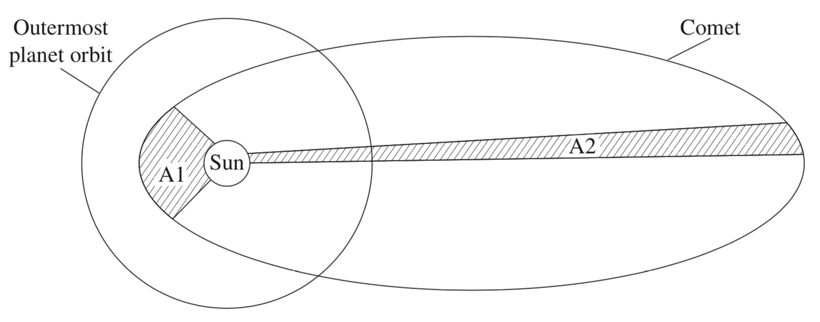6 (a) The resultant magnetic field patten is shown below.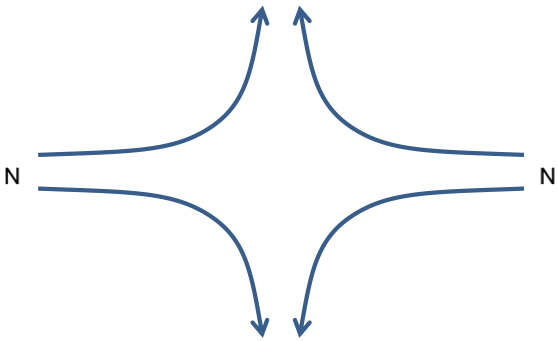(b) $B = 7.5 x 10^{-5}T$ 7 (a) End A is negative and End B is positive.(b) Bar is moving across a uniform magnetic field. Due to its relative motion, charges in the bar are moving perpendicular to the uniform magnetic field. Therefore, charges experience magnetic force resulting in electrons being separated from the positive charges. Hence a potential difference is setup known as emf – electromotive force.(c)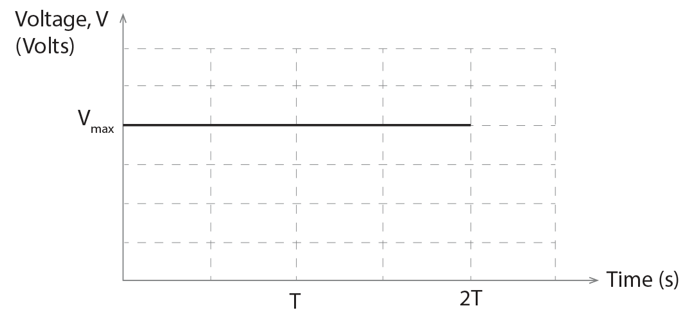8 (a) To determine the change in magnetic flux, we must first calculate the change in magnetic field strength from the graph.Step 1: Calculate the change in magnetic field strength over the 1.0 s period.$\Delta B= 0.8-(-0.5) = 1.3 T$Step 2: Calculate the change in magnetic flux$\Delta \Phi = \Delta B_\perp A=1.3 \times \pi (0.02)^2 =0.00163 \ Wb$ (b) Calculate the induced emf using the Faraday’s law of induction.$\varepsilon = -N \dfrac{\Delta \Phi}{\Delta t}= -100\dfrac{0.00163}{1.0} = - 0.163 \ V$ 9 $$d\sinθ = mλ \\ d \frac{\Delta x}{L} = m \lambda \\ \Delta x = \frac{m \lambda L}{d}$$Decreasing the slit separation (d) will increase Δx.Increasing the distance between the double slits and the screen (L) will increase Δx. 10 IC : IA  = 1 : 4 11 The flowchart below outlines the process undertaken by Fizeau for determining the speed of light.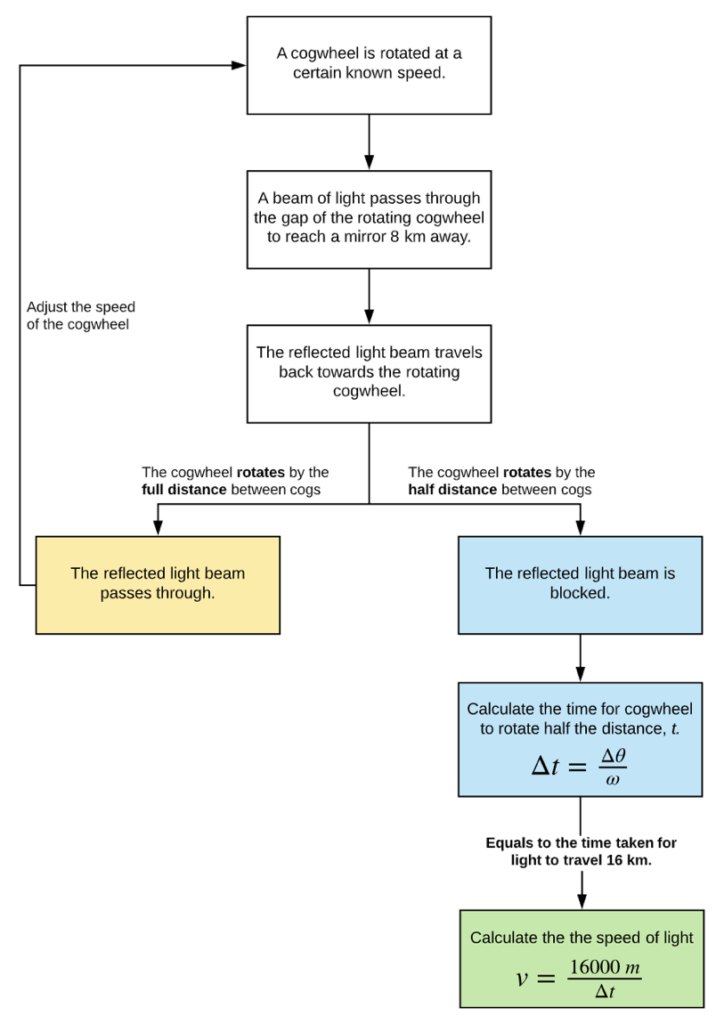12 Spectrum A: Incandescent globe is considered a blackbody and produces a blackbody spectrum.Spectrum B: Mercury vapour lamp produces discrete, specific wavelengths of light. It does not produce a continuous spectrum 13 (a) Star A has narrow absorption lines similar to the discharge tube and shows no signs of Doppler broadening, hence it is not rotating significantly (low or zero rotational velocity).Star B has wide absorption lines arising from Doppler broadening, hence must be rotating at higher speed.(b) Light from the star will be shifted as a result of the Doppler effect and its motion relative to Earth. A blueshift indicates the star moves towards Earth and a redshift indicates the star moves away from Earth. The amount of shift indicates the speed. 14 (a) The times on each clock were different as each clock experienced a different amount of time dilation. The eastwards moving clock recorded a shorter time than the ground clock. The westwards moving clock recorded a longer time than the ground clock.(b) The clocks travel at different speeds relative to the Earth’s axis and experience different amounts of time dilation. Hence they will be showing a different time for the duration of the experiment. The experimental results were consistent with the predictions of relativity. 15 (a) Use the flowchart below to describe the method.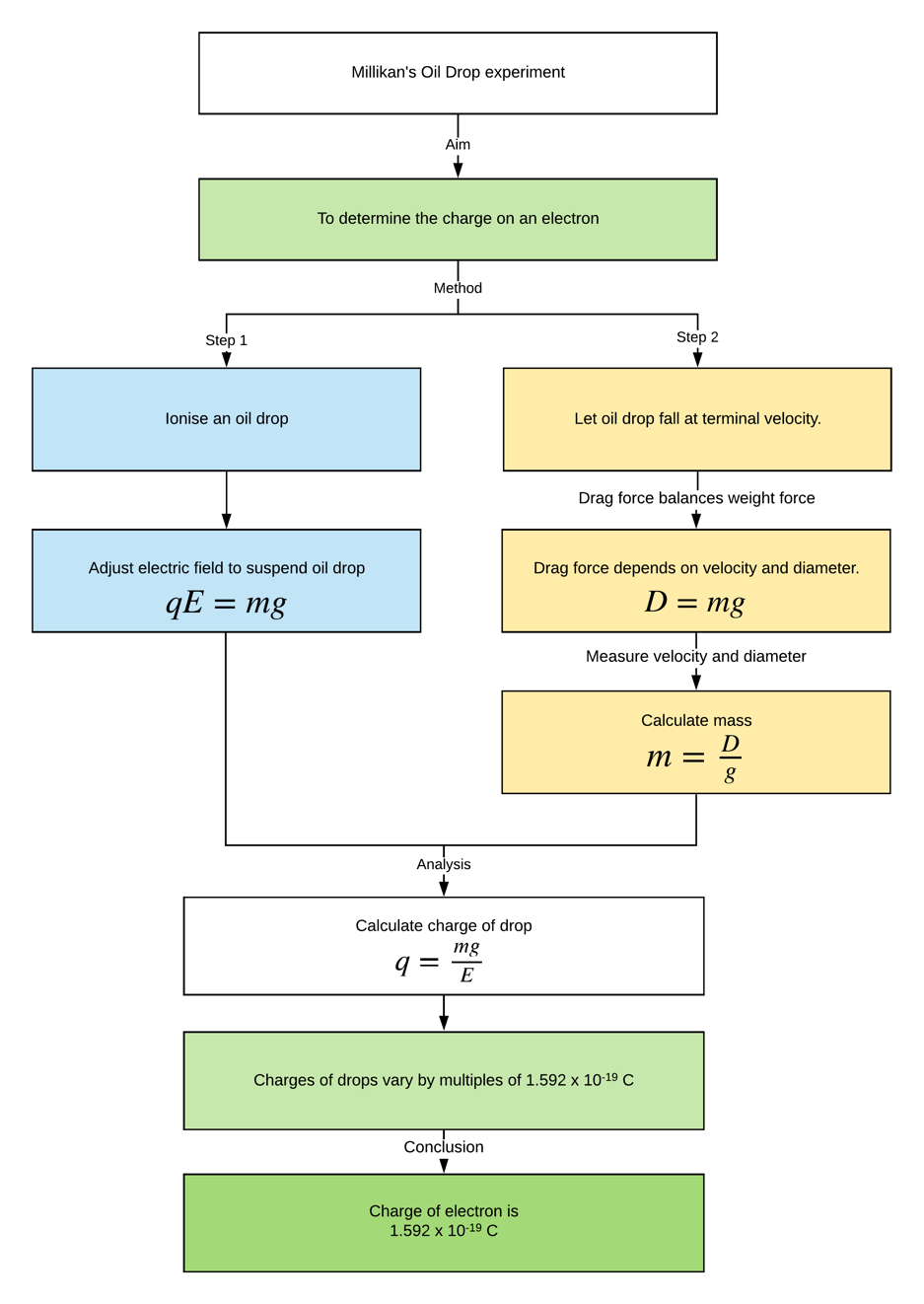(b) Charge of a particle is quantised. 16 The de Broglie-Bohr model of the atom had electrons orbiting as one dimensional standing waves in circular orbits. Schrodinger’s wave equation extended de Broglie’s proposal that particles have a wavelength and nature to fully characterise the waveform of particles in three dimensional space.When solved for  electrons around a nucleus, Schrodinger’s equation shows that electron waveforms are 3D standing waves of different shapes and energies.The resulting quantum model of the atom is thus 3D, and describes the structure, energies, transitions, and interactions electrons can have, properly accounting for element structure, line spectral details, and chemical reactions. 17 (a) 6 hours(b) λ = 0.116 hour-1 18 Cepheid variable stars were seen to pulsate in brightness with consistent period. By using parallax to measure the distance to nearby Cepheids, their luminosity could be calculated. It was found that there was a clear relationship between the luminosity of a Cepheid and its period of pulsation. This information was used when very distant Cepheids were observed. Their observed period of pulsation was used to determine their luminosity by the relationship, and with luminosity then known their brightness was observed to then determine their distance.Meanwhile Hubble had observations of the spectrum of light from the same distant galaxies in which the Cepheids were observed. The spectrum shown known atomic lines but redshifted, indicating a recessional speed. Hubble thus knew the distance from which these redshift and recessional velocities were. Hubble plotted the speed from the redshift against the distance. He found a linear relationship. This was a very strong trend and independent of direction (isotropic). This is what would be observed in an expanding universe, and would be very unlikely to be observed in a universe that wasn’t expanding. It was thus very strong observational proof that the universe is expanding. 19 (a) Star C: It has the largest luminosity and hence produces the greatest amount of light.(b)Star C: It has the highest colour index, so the reddest colour and lowest surface temperature. Star A is a protostar. 20 (a) Gravitational force compresses and heats a cloud of gas until temperature and pressure are high enough to start hydrogen core fusion.(b) Star X. A higher mass main sequence star is more luminous and hotter than a lower mass main sequence star.(c) Higher pressure and temperature mean much higher fusion rate. The star uses its larger amount of fuel much quicker.(d) Red giant:Lower surface temperaturehigher luminosityFusion of helium to produce carbonWhite dwarf:Higher surface temperaturelower luminosityNo nuclear fusion

Get free access to 1000+ exam style questions written by expert HSC teachers for the new syllabus. Discover your strengths and weakness with instant feedback on your quizzes.